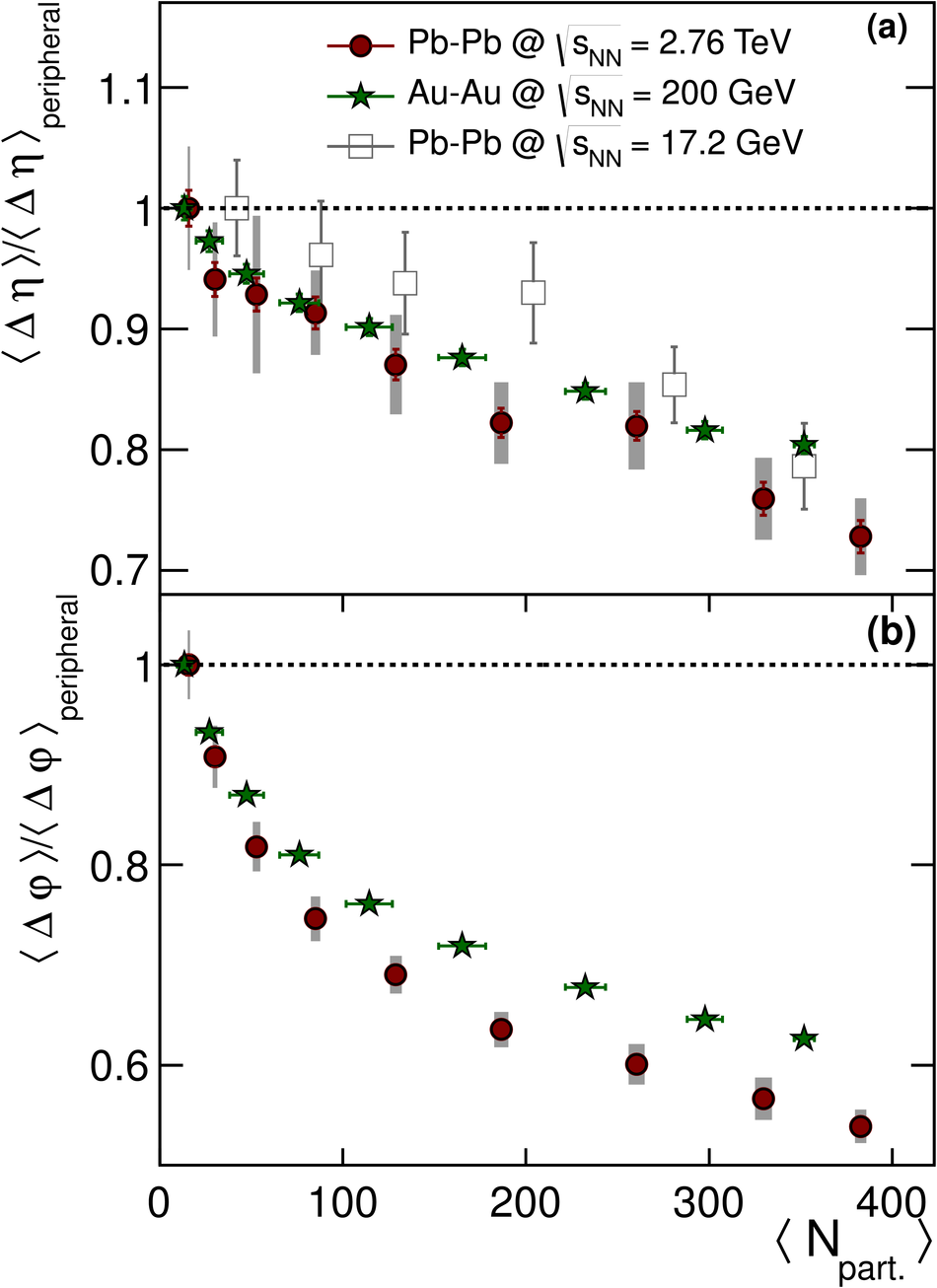# Charge correlations using the balance function in Pb-Pb collisions at $\sNN=2.76$ TeV

In high-energy heavy-ion collisions, the correlations between the emitted particles can be used as a probe to gain insight into the charge creation mechanisms. In this article, we report the first results of such studies using the electric charge balance function in the relative pseudorapidity $\Deta$ and azimuthal angle $\Dphi$ in Pb-Pb collisions at $\sNN=2.76$ TeV with the ALICE detector at the Large Hadron Collider. The width of the balance function decreases with growing centrality (i.e. for more central collisions) in both projections. This centrality dependence is not reproduced by HIJING, while AMPT, a model which incorporates strings and parton rescattering, exhibits qualitative agreement with the measured correlations in $\Dphi$ but fails to describe the correlations in $\Deta$. A thermal blast wave model incorporating local charge conservation and tuned to describe the $\pT$ spectra and $v_2$ measurements reported by ALICE, is used to fit the centrality dependence of the width of the balance function and to extract the average separation of balancing charges at freeze-out. The comparison of our results with measurements at lower energies reveals an ordering with $\sNN$: the balance functions become narrower with increasing energy for all centralities. This is consistent with the effect of larger radial flow at the LHC energies but also with the late stage creation scenario of balancing charges. However, the relative decrease of the balance function widths in $\Deta$ and $\Dphi$ with centrality from the highest SPS to the LHC energy exhibits only small differences. This observation cannot be interpreted solely within the framework where the majority of the charge is produced at a later stage in the evolution of the heavy-ion collision.

Figures

## Figure 1

 The correction factor $f_{+-}(\Delta\eta,\Delta\varphi)$ for the 5$\%$ most central Pb--Pb collisions, extracted from the single particle tracking efficiencies $\varepsilon(\eta,\varphi,p_{\rm T})$ and the acceptance terms $\alpha(\Delta\eta,\Delta\varphi)$ (see text for details).## Figure 2

 Balance function as a function of $\Deta$ for different centrality classes: 0-5% (a), 30-40% (b) and 70-80%. Mixed events results, not corrected for the detector effects, are shown by open squares. See text for details.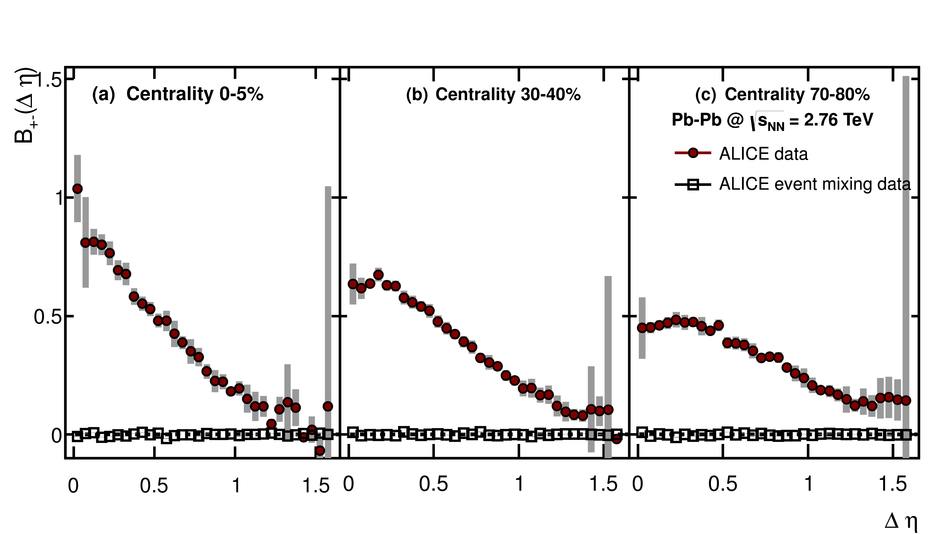## Figure 3

 Balance function as a function of $\Dphi$ for different centrality classes: 0-5% (a), 30-40% (b) and 70-80%. Mixed events results, not corrected for the detector effects, are shown by open squares. See text for details.## Figure 4

 The centrality dependence of the width of the balance function $\langle \Deta \rangle$ and $\langle \Dphi \rangle$, for the correlations studied in terms of the relative pseudorapidity and the relative azimuthal angle, respectively. The data points are compared to the predictions from HIJING, and AMPT.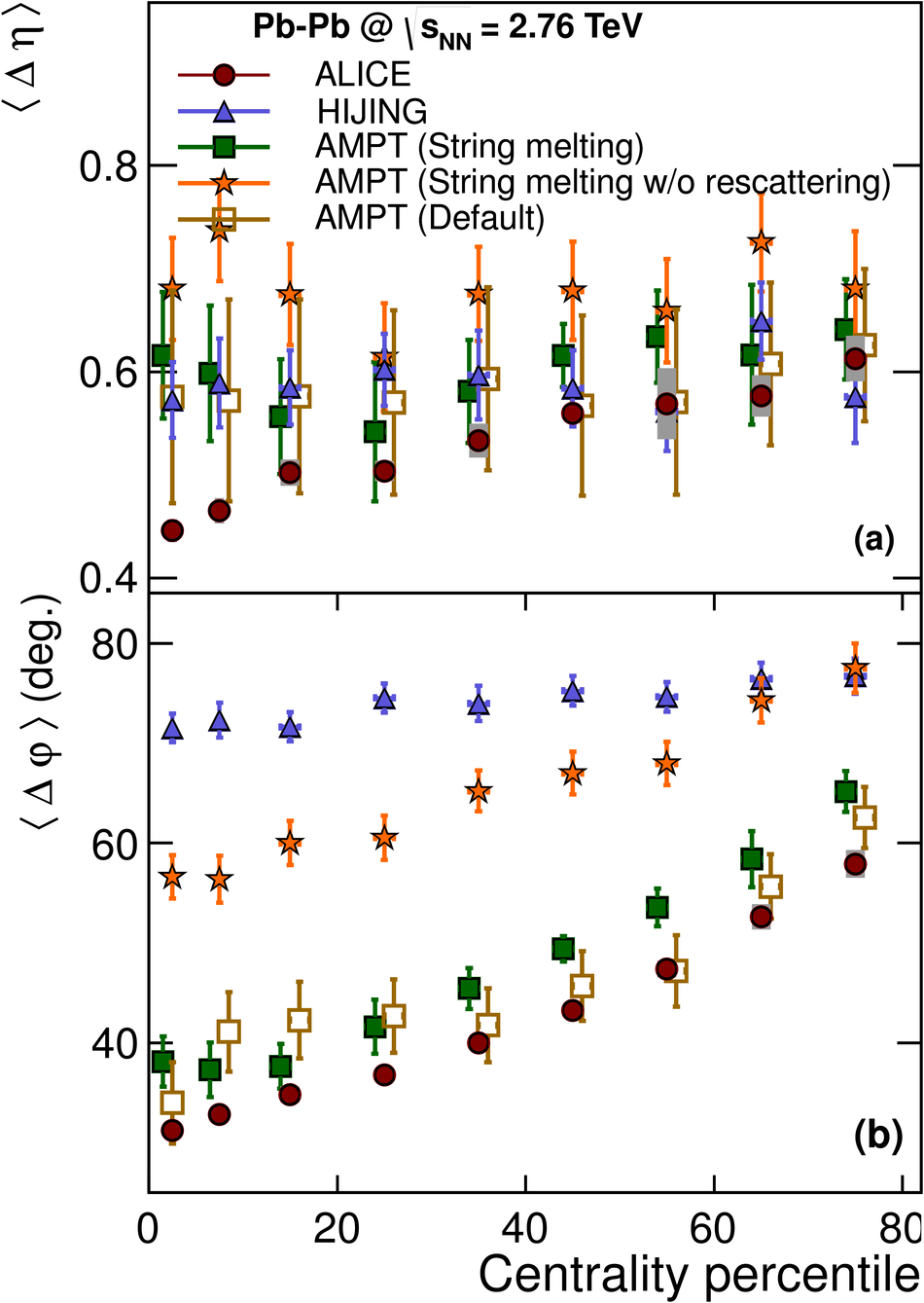## Figure 5

 The balance functions for the 5% most central Pb-Pb collisions measured by ALICE as a function of the relative pseudorapidity (a) and the relative azimuthal angle (b). The experimental points are compared to predictions from HIJING, AMPT and from a thermal blast wave model.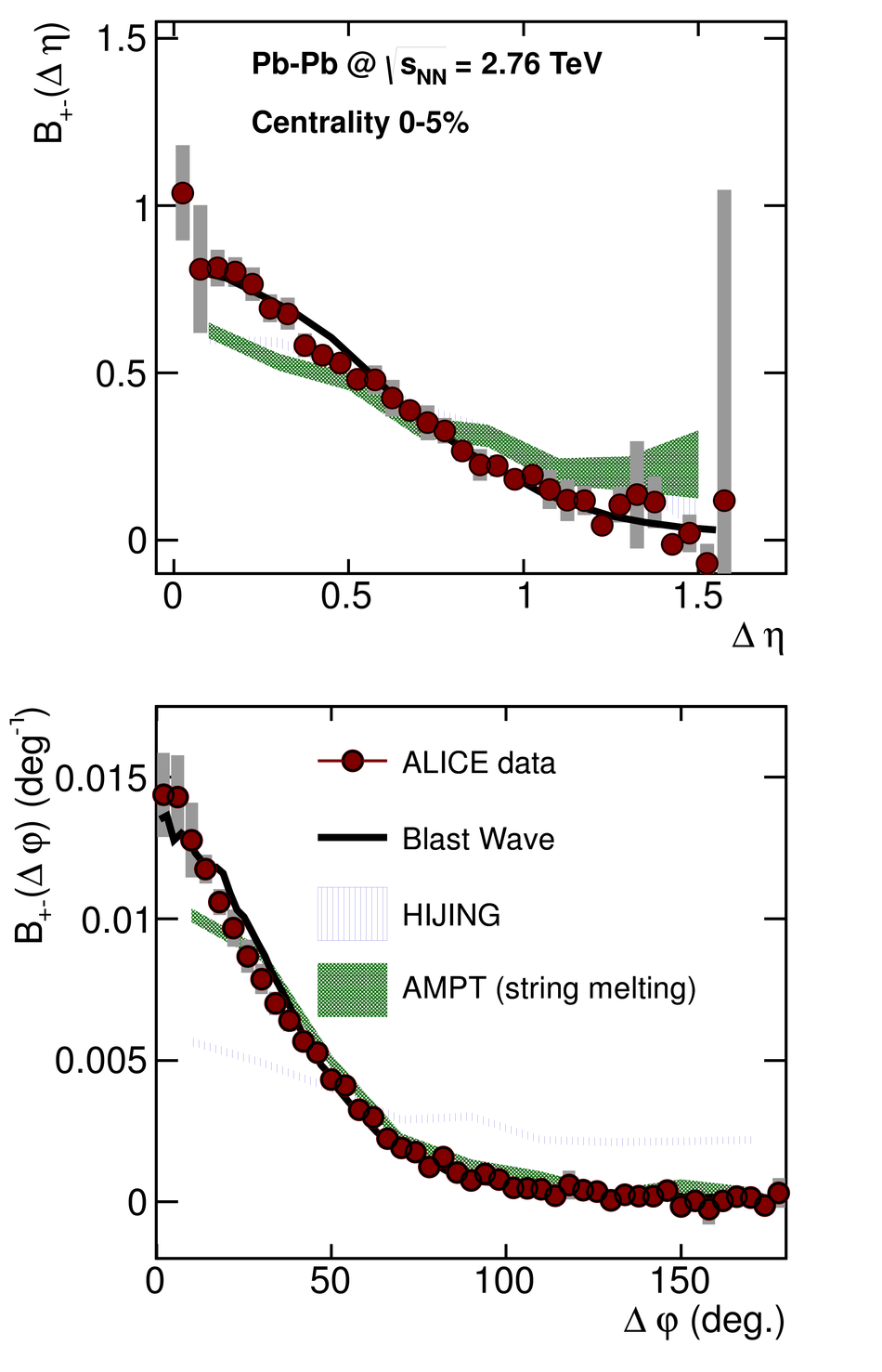## Figure 6

 The centrality dependence of the balance function width $\langle \Deta \rangle$ (a) and $\langle \Dphi \rangle$ (b). The ALICE points are compared to results from STAR. The STAR results have been corrected for the finite acceptance.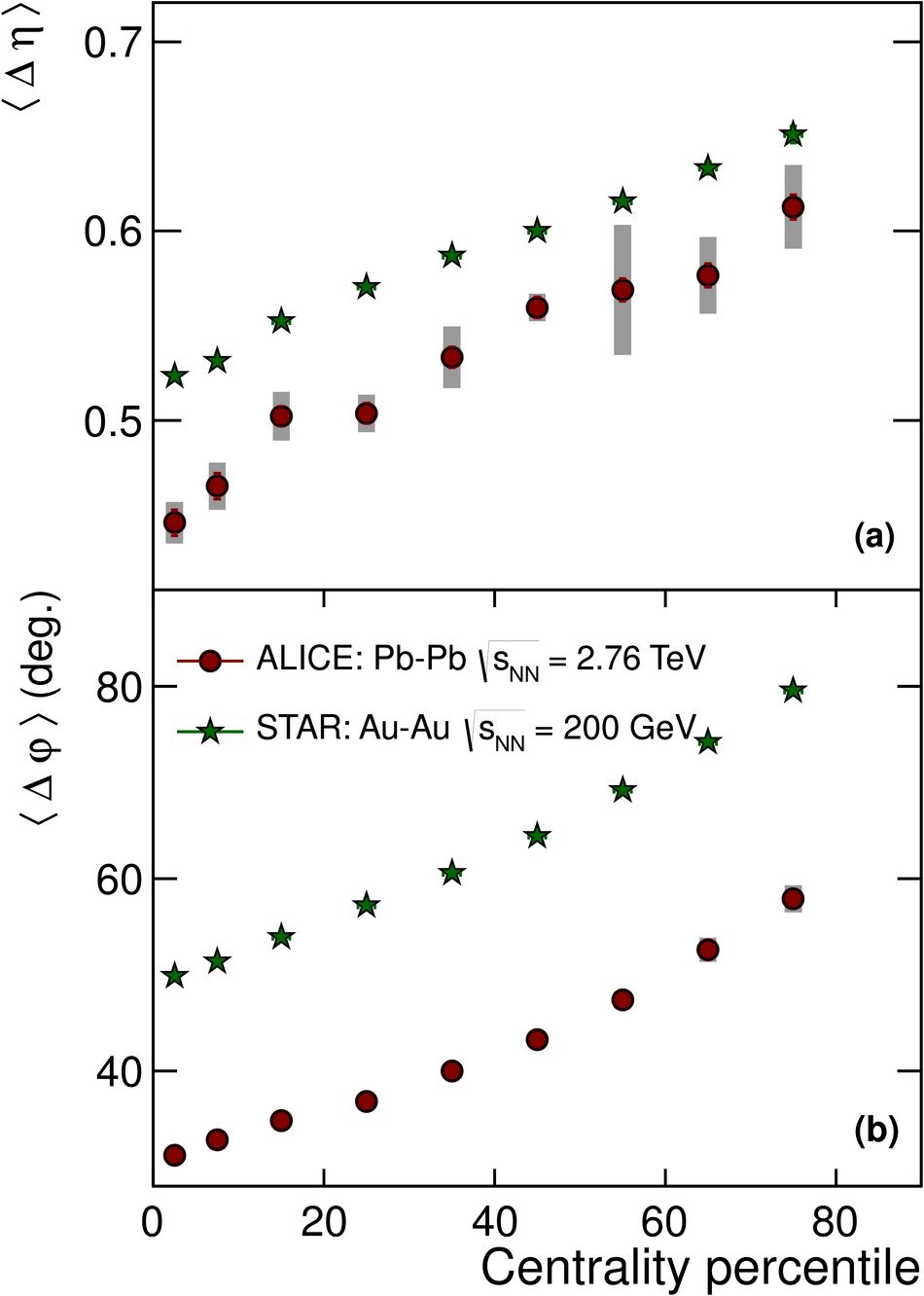## Figure 7

 The centrality dependence of the relative decrease of the width of the balance function in the relative pseudorapidity (a) and relative azimuthal angle (b). The ALICE points are compared to results for the highest SPS and RHIC energies.